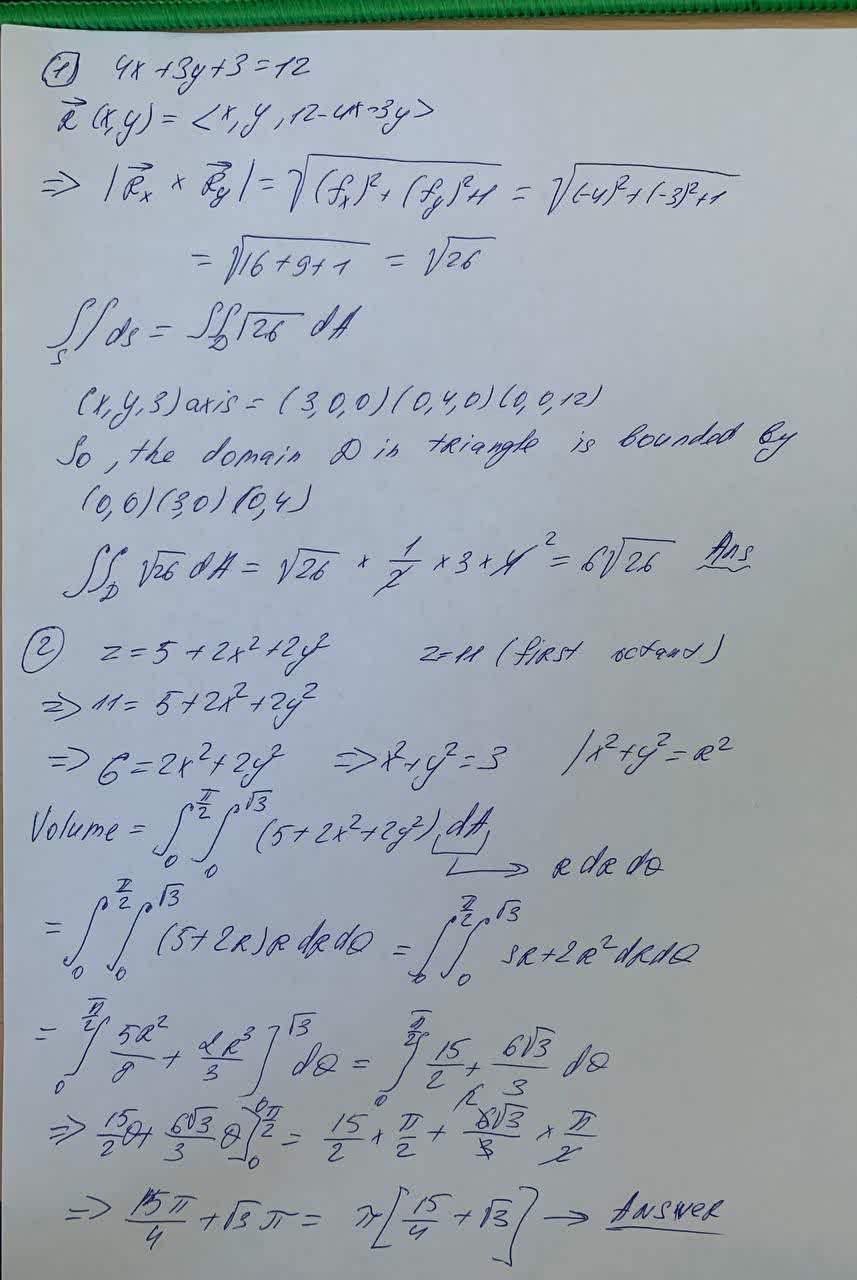Question# Find the area of the part of the plane. 4x + 3y + z = 12. that lies in the first octant.Use polar coordinates to find the volume of the given solid.

Parametric equations
ANSWERED1) Find the area of the part of the plane
$$4x + 3y + z = 12$$
that lies in the first octant.
2) Use polar coordinates to find the volume of the given solid.
Bounded by the paraboloid $$\displaystyle{z}={5}+{2}{x}^{{2}}+{2}{y}^{{2}}$$ and the plane z = 11 in the first octant